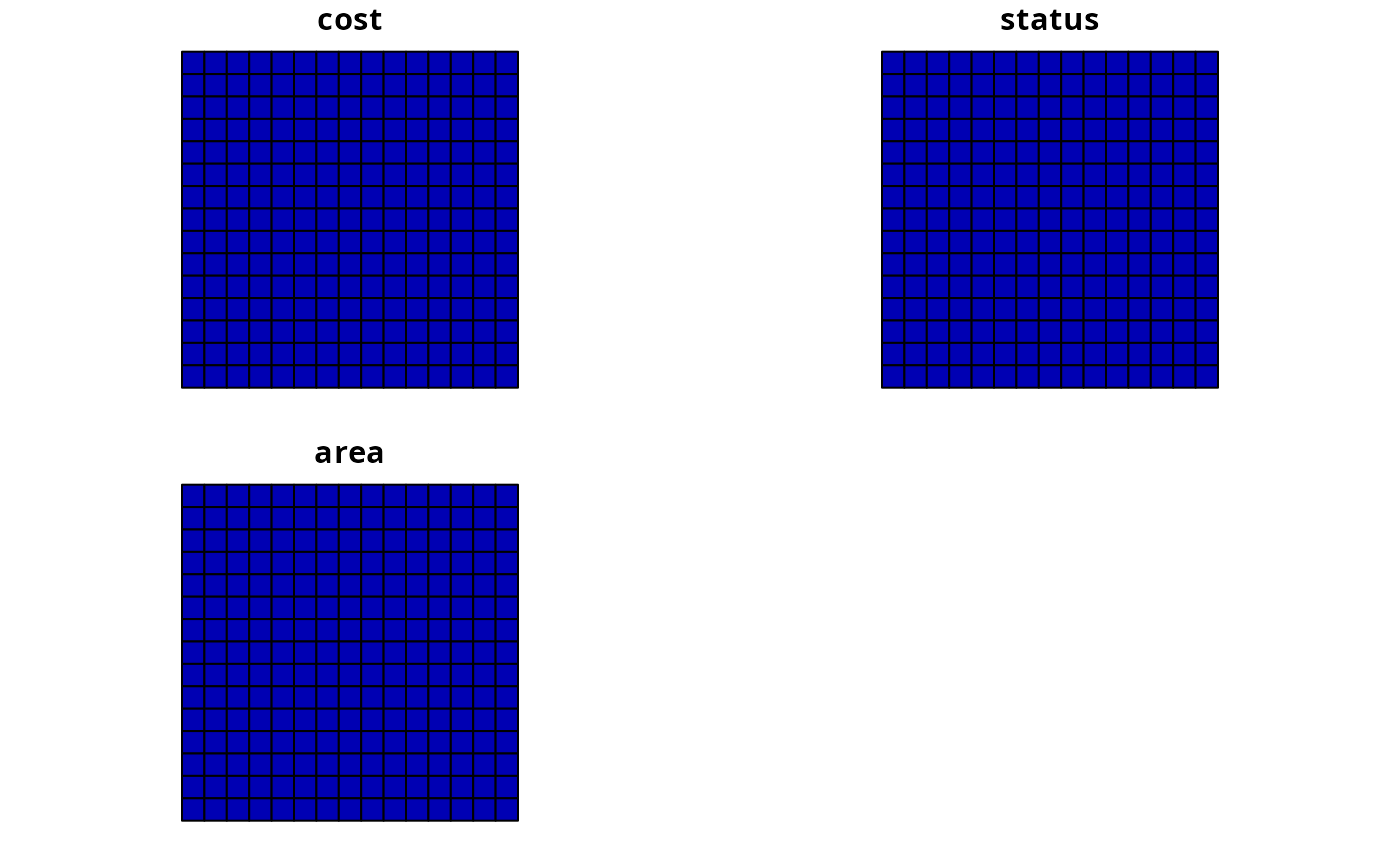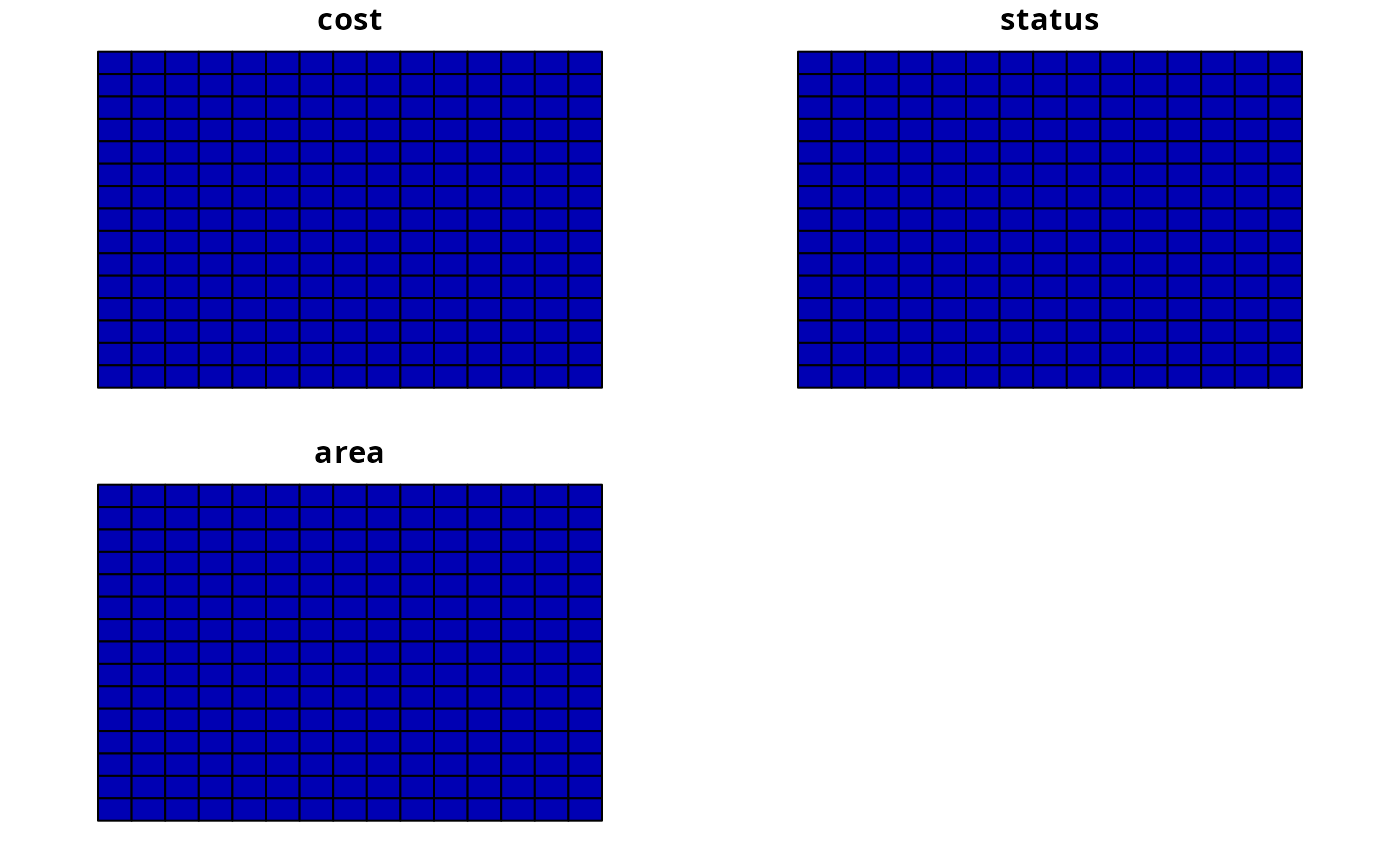This function simulates planning units for RAP.

sim.pus(
n,
xmn = -sqrt(n)/2,
xmx = sqrt(n)/2,
ymn = -sqrt(n)/2,
ymx = sqrt(n)/2
)

## Arguments

n

integer number of planning units. Note sqrt(n) must yield a valid number.

xmn

numeric value for minimum x-coordinate.

xmx

numeric value for maximum x-coordinate.

ymn

numeric value for minimum y-coordinate.

ymx

numeric value for maximum y-coordinate.

## Value

sf::st_as_sf() with planning units.

## Details

Square planning units are generated in the shape of a square. Default coordinate arguments are such that the planning units will be centered at origin. The data slot contains an "id" (integer), "cost" (numeric), "status" (integer), and "area" (numeric).

## Examples

# \dontrun{
# generate 225 square planning units arranged in a square
# with 1 unit height / width
x <- sim.pus(225)

# generate 225 rectangular pus arranged in a square
y <- sim.pus(225, xmn = -5, xmx = 10, ymn = -5, ymx = 5)
par(mfrow = c(1, 2))
plot(x, main = "x")plot(y, main = "y")par(mfrow = c(1, 1))
# }